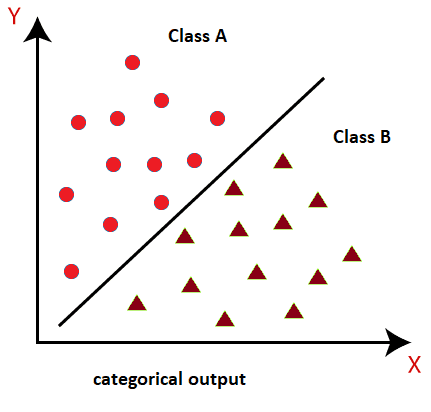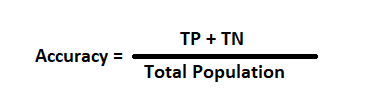# Classification Algorithm in Machine Learning

In this page we will learn Classification Algorithm in Machine Learning, What is the Classification Algorithm in Machine Learning?, Learners in Classification Problems, Types of ML Classification Algorithms, Evaluating a Classification model, Log Loss or Cross-Entropy Loss, Use cases of Classification Algorithms.

As we all know, the Regression and Classification Algorithms are two types of Supervised Machine Learning algorithms. We predicted the output for continuous values using Regression techniques, but we require Classification methods to predict categorical values.

## What is the Classification Algorithm in Machine Learning?

The Classification algorithm is a Supervised Learning technique that uses training data to determine the category of new observations. Classification is the process of a software learning from a dataset or observations and then classifying fresh observations into one of several classes or groupings. Yes or No, 0 or 1, Spam or Not Spam, cat or dog, and so forth. Targets/labels or categories are all terms that can be used to describe classes.

Unlike regression, Classification produces a category rather than a value, such as "Green or Blue," "fruit or animal," and so on. Because the Classification method is a Supervised learning technique, it uses labeled input data with corresponding output, which means it includes data that has been labeled.
In classification algorithm, a discrete output function(y) is mapped to input variable(x).

### y = f(x), where y = categorical output

Email Spam Detector is the greatest example of a machine learning classification method.
The fundamental purpose of a classification algorithm is to determine which category a dataset belongs to, and these algorithms are typically used to anticipate the output for categorical data.
The diagram below helps to understand classification methods. There are two classes in the diagram below: class A and class B. These classes share features in common with one another as well as differences from other classes.A classifier is the algorithm that performs the classification on a dataset. There are two sorts of classifications: classifications and classifications.

• Binary Classifier: This type of classifier is used when there are only two possible outputs to a classification task.
YES or NO, MALE or FEMALE, SPAM or NOT SPAM, CAT or DOG, and so on are some examples.
• Multi-class Classifier: A Multi-class Classifier is used when a classification task involves more than two outcomes.
Classifications of different sorts of crops, for example, or classifications of different types of music.

## Learners in Classification Problems:

There are two sorts of learners in classification problems: We

• Lazy Learners: A lazy learner saves the training dataset first and then waits for the test dataset. In the case of the lazy learner, classification is based on the most closely related data in the training dataset. Training takes less time, but projections take longer.
Case-based reasoning, for example, uses the K-NN method.
• Eager Learners: Before receiving a test dataset, Eager Learners create a classification model based on a training dataset. Eager Learners, in contrast to Lazy Learners, spend more time learning and less time predicting. Decision Trees, Nave Bayes, and ANN are some examples.

## Types of ML Classification Algorithms:

Classification Algorithms can be further divided into the Mainly two category:

• Linear Models
1. Logistic Regression
2. Support Vector Machines
• Non-linear Models
1. K-Nearest Neighbours
2. Kernel SVM
3. Naïve Bayes
4. Decision Tree Classification
5. Random Forest Classification

Note: We will learn the above algorithms in later chapters.

## Evaluating a Classification model:

It is vital to evaluate the performance of our model once it has been completed, whether it be a Classification or Regression model. So, we have the following options for testing a classification model:

## 1. Log Loss or Cross-Entropy Loss:

• It's used to measure how well a classifier performs when the result is a probability value between 0 and 1.
• The value of log loss should be close to 0 for a decent binary Classification model.
• If the anticipated value differs from the actual value, the value of log loss rises.
• The lower the log loss, the more accurate the model is.
• Cross-entropy can be determined for binary classification as follows:
?(ylog(p)+(1?y)log(1?p))
Where y= Actual output, p= predicted output.

## 2. Confusion Matrix:

• The confusion matrix generates a matrix/table as an output that describes the model's performance.
• The error matrix is another name for it.
• The matrix is made up of the results of the forecasts in a summary form, with a total number of correct and incorrect predictions. The matrix is shown in the table below:
Actual Positive Actual Negative
Predictive Positive True Positive False Positive
Predictive Negative False Negative True Negative## 3. AUC-ROC curve:

• The AUC refers for Area Under the Curve, and the ROC curve is for Receiver Operating Characteristics Curve.
• It's a graph that depicts the classification model's performance at various thresholds.
• The AUC-ROC Curve is used to visualize the performance of the multi-class classification model.
• TPR (True Positive Rate) is plotted on the Y-axis, while FPR (False Positive Rate) is plotted on the X-axis.

## Use cases of Classification Algorithms

Algorithms for classification can be employed in a variety of situations. Here are a few examples of how Classification Algorithms are used:

• Email Spam Detection
• Speech Recognition
• Identifications of Cancer tumor cells.
• Drugs Classification
• Biometric Identification, etc.